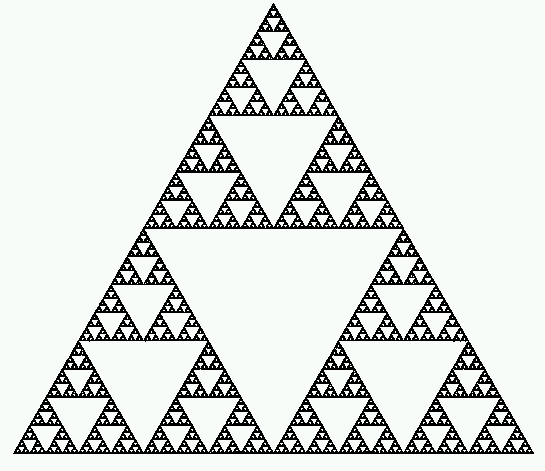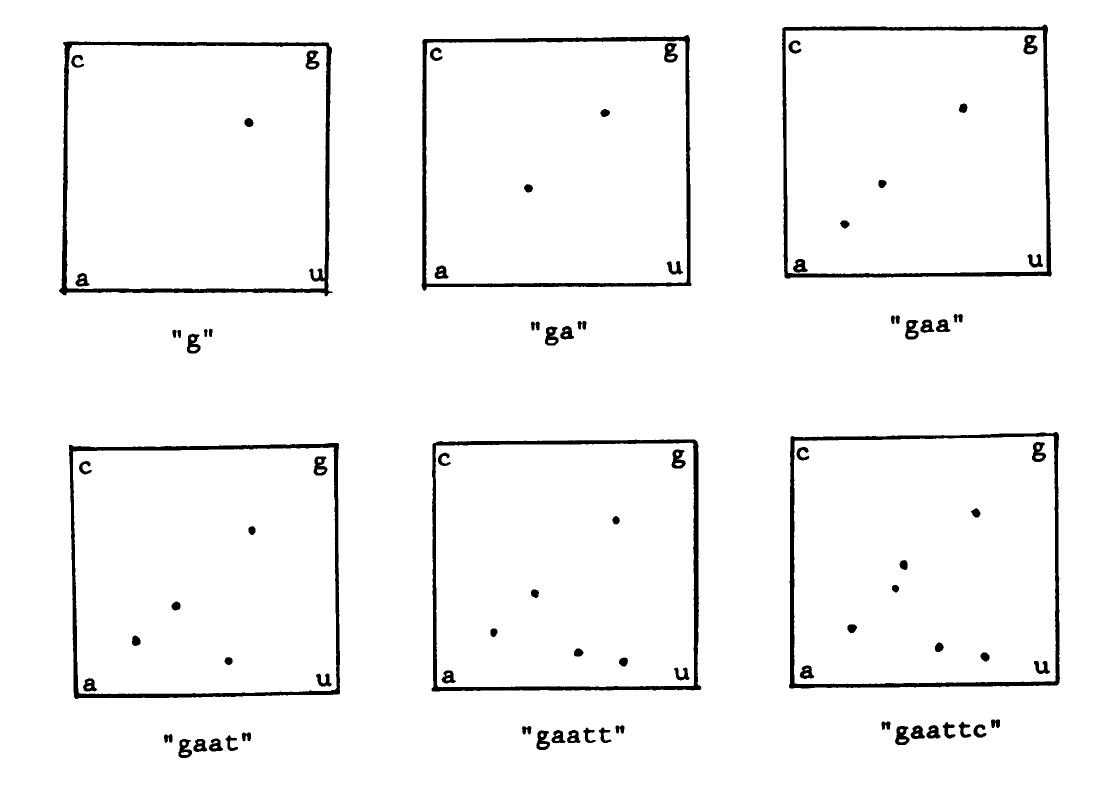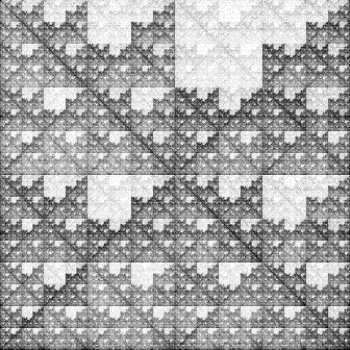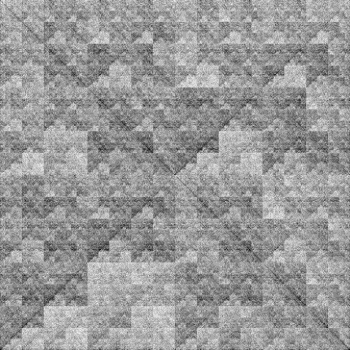Chaos Game Representations of DNA Sequences

This webpage offers the following options:

• If you wish to see the fractal pattern created by a sequence of your choice, click here.
• If you wish to compare the fractal pattern generated by a sequence of your choice with our library, click here.
• For more information on the chaos game and generating fractal patterns from genomic sequences, see below.

This website uses the principles involved with the Chaos Game to create fractal images of DNA sequences. In order to play the standard Chaos Game, you start with three vertexs, with each designated by two numbers. So the first vertex is 1 and 2, the second is 3 and 4, and the third is 5 and 6. Using the center of the triangle as your starting point, roll a die. Place another point halfway between the point you started with and the vertex that represents the number on the die. Then repeat this process using the new point as the initial point. If this process is repeated thousands of times, the image that results will be the Sierpinski Triangle, shown below.On this page, the Chaos Game is used slightly differently. Instead of using three vertices, this uses four vertices, each one corresponding to a different nucleotide. Also, instead of relying on randomized numbers, the program uses a DNA sequence to create the image. Shown below is the first six points of a sample sequence being plotted. Note that the starting point was the center of the square.Below are two sample fractal patterns created from chromosome sections of a mouse and A. fumigatus respectively. The mouse sequence used was 70.6 million nucleotides long and was found on contig 078297.6 of chromosome 1. The A. fumigatus was the entire 44.9 million nucleotide sequence contained on chromosome 1. Both images were created using a 3,000 by 3,000 matrix.

Mouse:A. fumigatus:Sources:

Deschavanne, Patrick, Alain Giron, Joseph Vilain, Guillaume Fagot, and Bernard Fertil. "Genomic Signature: Characterization and Classification of Species Assessed by Chaos Game Representation of Sequences". Mol. Biol. Evol. 16(10): 1391 - 1399. 1999.

Jeffrey, H. Joel. "Chaos Game Representation of Gene Structure." Nucleic Acids Research 18(8):2163 - 2170. 1990.